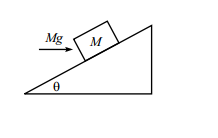# Block on InclineA block of mass $M$ rests on an fixed incline plane of angle $\theta$. A horizontal force of magnitude $Mg$ is applied on the block. If the friction force is large enough to keep the block at rest, for what value of $\theta$ in degrees is the normal force exerted by the plane on the block at its maximum?

×